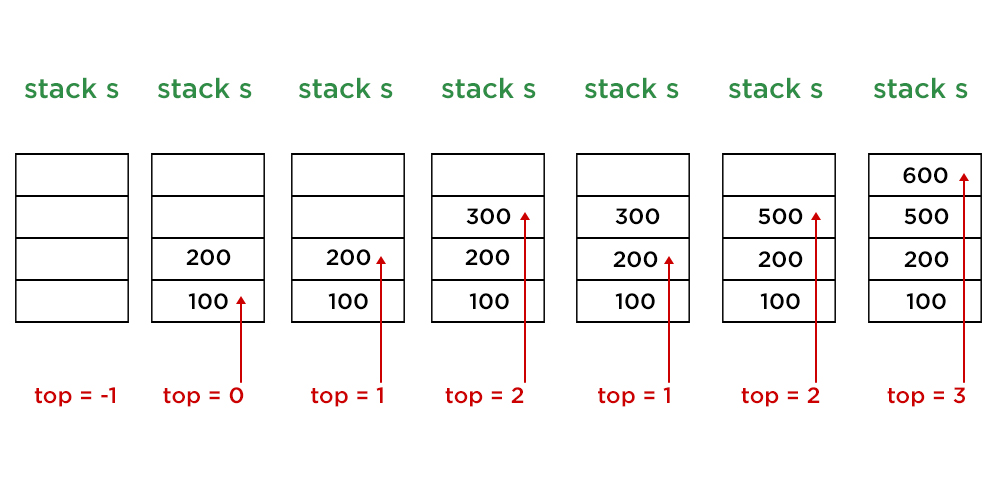Skip to content
Related Articles
How to Implement Stack in Java Using Array and Generics?
• Last Updated : 08 Jun, 2021

Stack is a linear Data Structure that is based on the LIFO concept (last in first out). Instead of only an Integer Stack, Stack can be of String, Character, or even Float type. There are 4 primary operations in the stack as follows:

1. push() Method adds element x to the stack.
2. pop() Method removes the last element of the stack.
3. top() Method returns the last element of the stack.
4. empty() Method returns whether the stack is empty or not.

Note: Time Complexity is of order 1 for all operations of the stack

Illustration:Stack 1

`let s = empty stack of Integer type with size 4`

Stack 2

`push (100) : top = top + 1 and s[top] = 100 `

Stack 3

`push (200) : top = top + 1 and s[top] = 200`

Stack 4

` push (300) : top = top + 1 and s[top] = 300`

Stack 5

`pop ( )  : top = top - 1`

Stack 6

`push (500) : top = top + 1 and s[top] = 500`

Stack 7

`push (600) : top = top + 1 and s[top] = 600`

Note:

```push (700) : top +1 == size of stack : Stack Overflow !
// Since top = 3 and size of stack  = 4, no more elements can be pushed```

Implementation:

Example

## Java

 `// Java Program to Implement Stack in Java Using Array and``// Generics` `// Importing input output classes``import` `java.io.*;``// Importing all utility classes``import` `java.util.*;` `// user defined class for generic stack``class` `stack {` `    ``// Empty array list``    ``ArrayList A;` `    ``// Default value of top variable when stack is empty``    ``int` `top = -``1``;` `    ``// Variable to store size of array``    ``int` `size;` `    ``// Constructor of this class``    ``// To initialize stack``    ``stack(``int` `size)``    ``{``        ``// Storing the value of size into global variable``        ``this``.size = size;` `        ``// Creating array of Size = size``        ``this``.A = ``new` `ArrayList(size);``    ``}` `    ``// Method 1``    ``// To push generic element into stack``    ``void` `push(T X)``    ``{``        ``// Checking if array is full``        ``if` `(top + ``1` `== size) {` `            ``// Display message when array is full``            ``System.out.println(``"Stack Overflow"``);``        ``}``        ``else` `{` `            ``// Increment top to go to next position``            ``top = top + ``1``;` `            ``// Over-writing existing element``            ``if` `(A.size() > top)``                ``A.set(top, X);` `            ``else` `                ``// Creating new element``                ``A.add(X);``        ``}``    ``}``    ``// Method 2``    ``// To return topmost element of stack``    ``T top()``    ``{``        ``// If stack is empty``        ``if` `(top == -``1``) {` `            ``// Display message when there are no elements in``            ``// the stack``            ``System.out.println(``"Stack Underflow"``);` `            ``return` `null``;``        ``}` `        ``// else elements are present so``        ``// return the topmost element``        ``else``            ``return` `A.get(top);``    ``}` `    ``// Method 3``    ``// To delete last element of stack``    ``void` `pop()``    ``{``        ``// If stack is empty``        ``if` `(top == -``1``) {` `            ``// Display message when there are no elements in``            ``// the stack``            ``System.out.println(``"Stack Underflow"``);``        ``}` `        ``else` `            ``// Delete the last element``            ``// by decrementing the top``            ``top--;``    ``}` `    ``// Method 4``    ``// To check if stack is empty or not``    ``boolean` `empty() { ``return` `top == -``1``; }` `    ``// Method 5``    ``// To print the stack``    ``// @Override``    ``public` `String toString()``    ``{` `        ``String Ans = ``""``;` `        ``for` `(``int` `i = ``0``; i < top; i++) {``            ``Ans += String.valueOf(A.get(i)) + ``"->"``;``        ``}` `        ``Ans += String.valueOf(A.get(top));` `        ``return` `Ans;``    ``}``}``// Main Class``public` `class` `GFG {` `    ``// main driver method``    ``public` `static` `void` `main(String[] args)``    ``{` `        ``// Integer Stack` `        ``// Creating an object of Stack class``        ``// Declaring objects of Integer type``        ``stack s1 = ``new` `stack<>(``3``);` `        ``// Pushing elements to integer stack - s1` `        ``// Element 1 - 10``        ``s1.push(``10``);``        ``// Element 2 - 20``        ``s1.push(``20``);``        ``// Element 3 - 30``        ``s1.push(``30``);` `        ``// Print the stack elements after pushing the``        ``// elements``        ``System.out.println(``            ``"s1 after pushing 10, 20 and 30 :\n"` `+ s1);` `        ``// Now, pop from stack s1``        ``s1.pop();` `        ``// Print the stack elements after poping few``        ``// element/s``        ``System.out.println(``"s1 after pop :\n"` `+ s1);` `        ``// String Stack` `        ``// Creating an object of Stack class``        ``// Declaring objects of Integer type``        ``stack s2 = ``new` `stack<>(``3``);` `        ``// Pushing elements to string stack - s2` `        ``// Element 1 - hello``        ``s2.push(``"hello"``);``        ``// Element 2 - world``        ``s2.push(``"world"``);``        ``// Element 3 - java``        ``s2.push(``"java"``);` `        ``// Print string stack after pushing above string``        ``// elements``        ``System.out.println(``            ``"\ns2 after pushing 3 elements :\n"` `+ s2);` `        ``System.out.println(``            ``"s2 after pushing 4th element :"``);` `        ``// Pushing another element to above stack` `        ``// Element 4 - GFG``        ``s2.push(``"GFG"``);` `        ``// Float stack` `        ``// Creating an object of Stack class``        ``// Declaring objects of Integer type``        ``stack s3 = ``new` `stack<>(``2``);` `        ``// Pushing elements to float stack - s3` `        ``// Element 1 - 100.0``        ``s3.push(``100``.0f);``        ``// Element 2 - 200.0``        ``s3.push(``200``.0f);` `        ``// Print string stack after pushing above float``        ``// elements``        ``System.out.println(``            ``"\ns3 after pushing 2 elements :\n"` `+ s3);` `        ``// Print and display top element of stack s3``        ``System.out.println(``"top element of s3:\n"``                           ``+ s3.top());``    ``}``}`

Output
```s1 after pushing 10, 20 and 30 :
10->20->30
s1 after pop :
10->20

s2 after pushing 3 elements :
hello->world->java
s2 after pushing 4th element :
Stack Overflow

s3 after pushing 2 elements :
100.0->200.0
top element of s3:
200.0```

Attention reader! Don’t stop learning now. Get hold of all the important Java Foundation and Collections concepts with the Fundamentals of Java and Java Collections Course at a student-friendly price and become industry ready. To complete your preparation from learning a language to DS Algo and many more,  please refer Complete Interview Preparation Course.

My Personal Notes arrow_drop_up